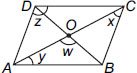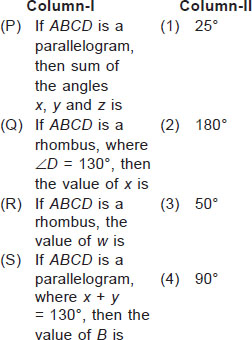# User ForumSubject :IMO    Class : Class 9

By using a given figure of quadrilateral ABCD, match the following:A (P)→(1), (Q)→(2), (R)→(3), (S)→(4)
B (P)→(2), (Q)→(1), (R)→(4), (S)→(3)
C (P)→(3), (Q)→(1), (R)→(2), (S)→(4)
D (P)→(4), (Q)→(3), (R)→(1), (S)→(2)

According to logic and also the solution, answer should be option B but the answer is given as option C. So can anyone explain?

## Ans 1:

Class : Class 9
Option B is correct

Class : Class 9
B option as

## Ans 3:

Class : Class 9
B is correct option.